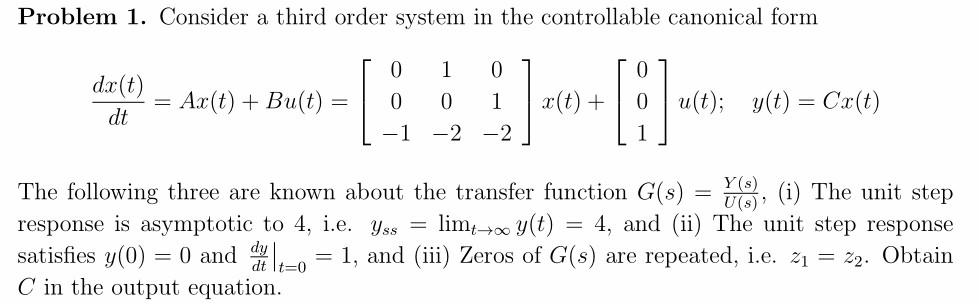# Question Solved1 AnswerProblem 1. Consider a third order system in the controllable canonical form dx(t) dt Ar(t) + Bu(t) x(t) + 0 1 -2 -2 u(t); y(t) = Cx(t) Y( = The following three are known about the transfer function G(s) VE), (i) The unit step response is asymptotic to 4, i.e. Yss = limt y(t) 4, and (ii) The unit step response satisfies y(0) = 0 and die 2–0 = 1, and (iii) Zeros of G(s) are repeated, i.e. z1 = 22. Obtain C in the output equation.Transcribed Image Text: Problem 1. Consider a third order system in the controllable canonical form dx(t) dt Ar(t) + Bu(t) x(t) + 0 1 -2 -2 u(t); y(t) = Cx(t) Y( = The following three are known about the transfer function G(s) VE), (i) The unit step response is asymptotic to 4, i.e. Yss = limt y(t) 4, and (ii) The unit step response satisfies y(0) = 0 and die 2–0 = 1, and (iii) Zeros of G(s) are repeated, i.e. z1 = 22. Obtain C in the output equation.
More
Transcribed Image Text: Problem 1. Consider a third order system in the controllable canonical form dx(t) dt Ar(t) + Bu(t) x(t) + 0 1 -2 -2 u(t); y(t) = Cx(t) Y( = The following three are known about the transfer function G(s) VE), (i) The unit step response is asymptotic to 4, i.e. Yss = limt y(t) 4, and (ii) The unit step response satisfies y(0) = 0 and die 2–0 = 1, and (iii) Zeros of G(s) are repeated, i.e. z1 = 22. Obtain C in the output equation.